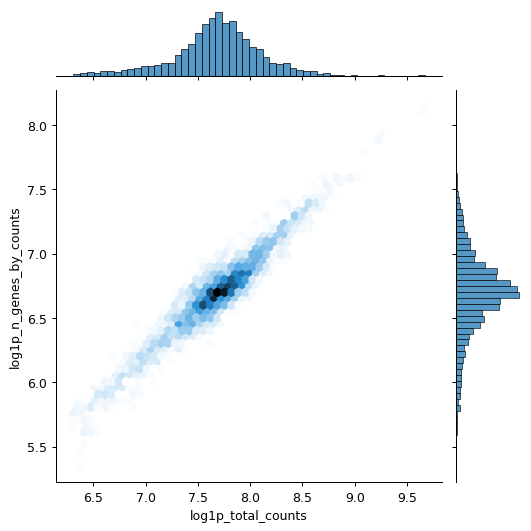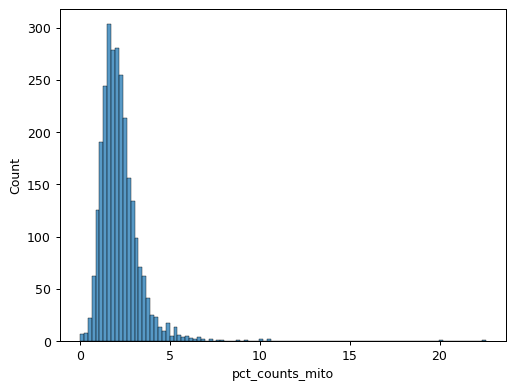# scanpy.pp.calculate_qc_metrics¶

`scanpy.pp.``calculate_qc_metrics`(adata, *, expr_type='counts', var_type='genes', qc_vars=(), percent_top=(50, 100, 200, 500), layer=None, use_raw=False, inplace=False, log1p=True, parallel=None)

Calculate quality control metrics.

Calculates a number of qc metrics for an AnnData object, see section `Returns` for specifics. Largely based on `calculateQCMetrics` from scater [McCarthy17]. Currently is most efficient on a sparse CSR or dense matrix.

Note that this method can take a while to compile on the first call. That result is then cached to disk to be used later.

Parameters
adata : `AnnData``AnnData`

Annotated data matrix.

expr_type : `str``str` (default: `'counts'`)

Name of kind of values in X.

var_type : `str``str` (default: `'genes'`)

The kind of thing the variables are.

qc_vars : (default: `()`)

Keys for boolean columns of `.var` which identify variables you could want to control for (e.g. “ERCC” or “mito”).

percent_top : (default: `(50, 100, 200, 500)`)

Which proportions of top genes to cover. If empty or `None` don’t calculate. Values are considered 1-indexed, `percent_top=` finds cumulative proportion to the 50th most expressed gene.

layer : (default: `None`)

If provided, use `adata.layers[layer]` for expression values instead of `adata.X`.

use_raw : `bool``bool` (default: `False`)

If True, use `adata.raw.X` for expression values instead of `adata.X`.

inplace : `bool``bool` (default: `False`)

Whether to place calculated metrics in `adata`’s `.obs` and `.var`.

log1p : `bool``bool` (default: `True`)

Set to `False` to skip computing `log1p` transformed annotations.

Return type
Returns

Depending on `inplace` returns calculated metrics (as `DataFrame`) or updates `adata`’s `obs` and `var`.

Observation level metrics include:

`total_{var_type}_by_{expr_type}`

E.g. “total_genes_by_counts”. Number of genes with positive counts in a cell.

`total_{expr_type}`

E.g. “total_counts”. Total number of counts for a cell.

`pct_{expr_type}_in_top_{n}_{var_type}` – for `n` in `percent_top`

E.g. “pct_counts_in_top_50_genes”. Cumulative percentage of counts for 50 most expressed genes in a cell.

`total_{expr_type}_{qc_var}` – for `qc_var` in `qc_vars`

E.g. “total_counts_mito”. Total number of counts for variabes in `qc_vars`.

`pct_{expr_type}_{qc_var}` – for `qc_var` in `qc_vars`

E.g. “pct_counts_mito”. Proportion of total counts for a cell which are mitochondrial.

Variable level metrics include:

`total_{expr_type}`

E.g. “total_counts”. Sum of counts for a gene.

`n_genes_by_{expr_type}`

E.g. “n_genes_by_counts”. The number of genes with at least 1 count in a cell. Calculated for all cells.

`mean_{expr_type}`

E.g. “mean_counts”. Mean expression over all cells.

`n_cells_by_{expr_type}`

E.g. “n_cells_by_counts”. Number of cells this expression is measured in.

`pct_dropout_by_{expr_type}`

E.g. “pct_dropout_by_counts”. Percentage of cells this feature does not appear in.

Example

Calculate qc metrics for visualization.

```import scanpy as sc
import seaborn as sns

pbmc = sc.datasets.pbmc3k()
pbmc.var["mito"] = pbmc.var_names.str.startswith("MT-")
sc.pp.calculate_qc_metrics(pbmc, qc_vars=["mito"], inplace=True)
sns.jointplot(
data=pbmc.obs,
x="log1p_total_counts",
y="log1p_n_genes_by_counts",
kind="hex",
)
``````sns.histplot(pbmc.obs["pct_counts_mito"])
```